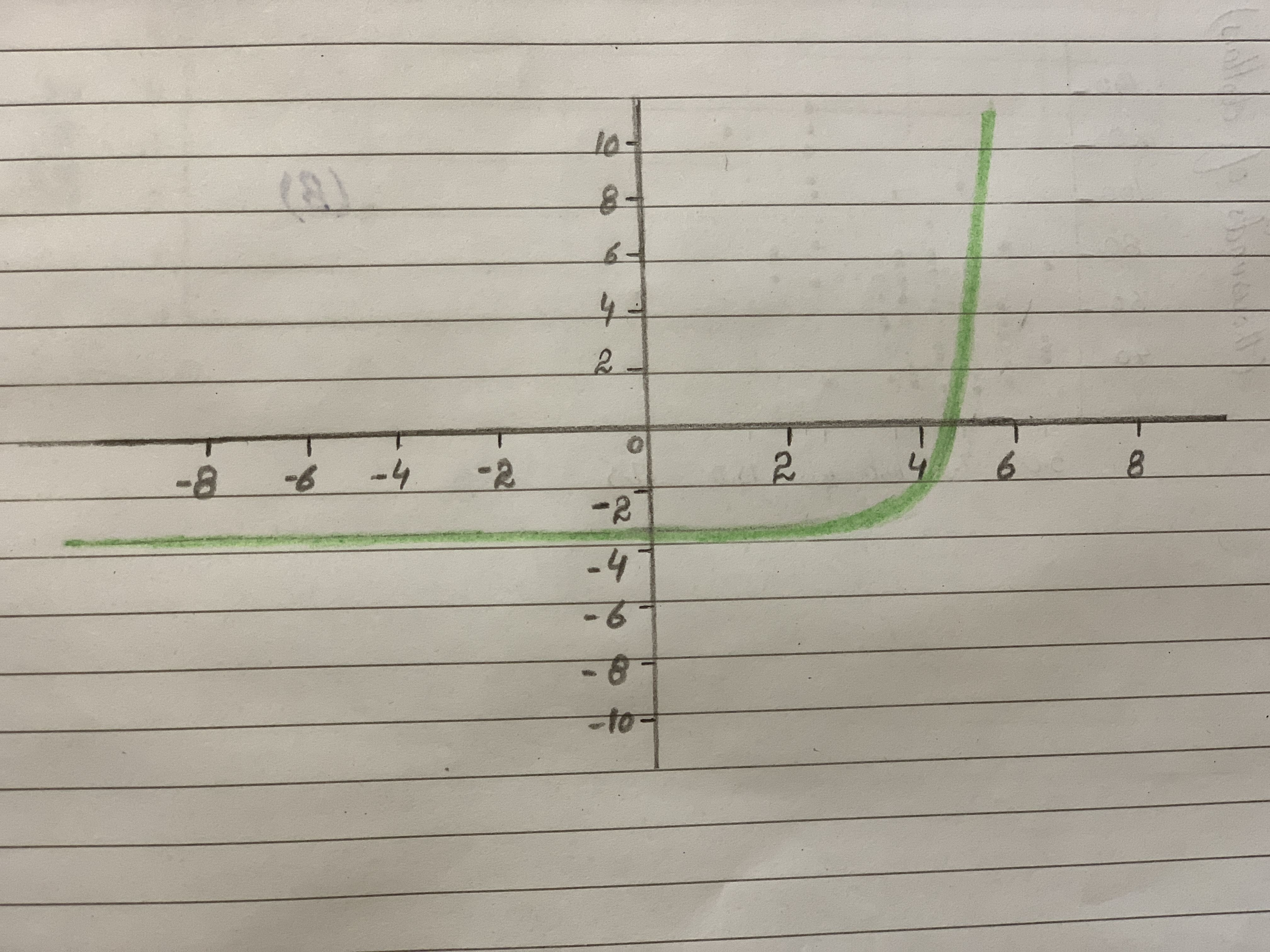# graph the following. be sure to include the ordered pairs, including any existing x-intercepts (logarithms) and y-intercept (exponentials) along with asymptotes. f(x)=2^(x-3)-3bobbie71G 2020-11-09 Answered
graph the following. be sure to include the ordered pairs, including any existing x-intercepts (logarithms) and y-intercept (exponentials) along with asymptotes.
$f\left(x\right)={2}^{\left(}x-3\right)-3$
You can still ask an expert for help

• Questions are typically answered in as fast as 30 minutes

Solve your problem for the price of one coffee

• Math expert for every subject
• Pay only if we can solve itdiskusje5

$f\left(x\right)={2}^{\left(}x-3\right)-3$
$=>y={2}^{\left(}x-3\right)-3$
$=>y+3={2}^{\left(}x-3\right)$
$=>log\left(y+3\right)=log{2}^{\left(}x-3\right)$
$=>log\left(y+3\right)=\left(x-3\right)\left(log2\right)$